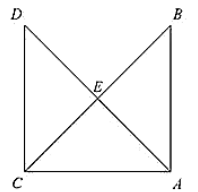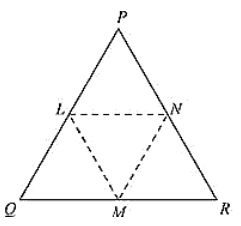×#### Thank you for registering.

One of our academic counsellors will contact you within 1 working day.

Click to Chat

1800-1023-196

+91-120-4616500

CART 0

• 0

MY CART (5)

Use Coupon: CART20 and get 20% off on all online Study Material

ITEM
DETAILS
MRP
DISCOUNT
FINAL PRICE
Total Price: Rs.

There are no items in this cart.
Continue Shopping• Complete JEE Main/Advanced Course and Test Series
• OFFERED PRICE: Rs. 15,900
• View Details

```Chapter 10: Congruent Triangles Exercise – 10.4

Question: 1

In figure, It is given that AB = CD and AD = BC. Prove that ΔADC ≅ ΔCBA.Solution:

Given that in the figure AB = CD and AD = BC.

We have to prove ΔADC ≅ ΔCBANow,

We have

AB = CD                     [Given]

And AC = AC             [Common side]

So, by SSS congruence criterion, we have

Hence proved

Question: 2

In a Δ PQR. IF PQ = QR and L, M and N are the mid-points of the sides PQ, QR and RP respectively. Prove that LN = MN.

Solution:

Given that in ΔPQR, PQ = QR and L, M and N are the mid-points of the sides PQ, QR and RP respectively

We have to prove LN = MN.Join L and M, M and N, N and L

We have PL = LQ, QM = MR and RN = NP

[Since, L, M and N are mid-points of Pp. QR and RP respectively]

And also PQ = QR

PL = LQ = QM = MR = PQ/2 = QR/2 ... (i) Using mid-point theorem,

We have

MN ∥ PQ and MN = PQ/2

MN = PL = LQ  ... (ii)

Similarly, we have

LN ∥ QR and LN = (1/2)QR

LN = QM = MR ... (iii)

From equation (i), (ii) and (iii), we have

PL = LQ = QM = MR = MN = LN

LN = MN
```### Course Features

• 728 Video Lectures
• Revision Notes
• Previous Year Papers
• Mind Map
• Study Planner
• NCERT Solutions
• Discussion Forum
• Test paper with Video Solution